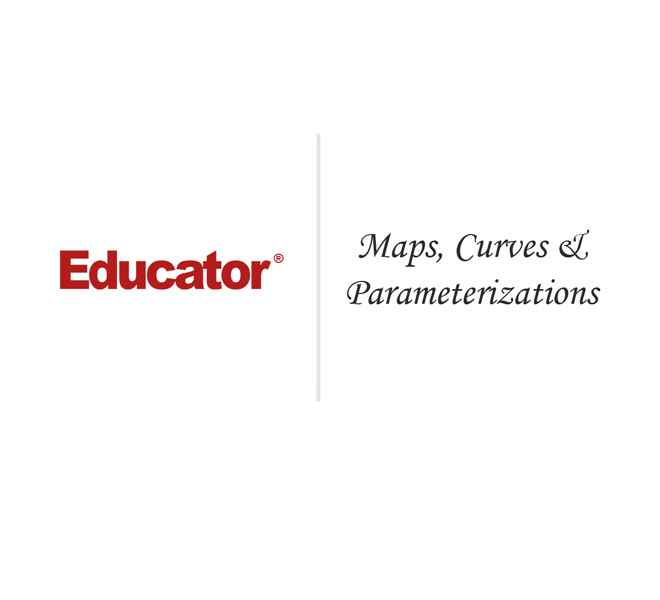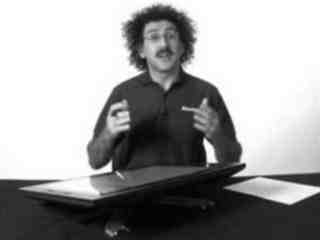Raffi Hovasapian

Maps, Curves & Parameterizations

Slide Duration:

Section 1: Vectors
Points & Vectors

28m 23s

Intro
0:00
Points and Vectors
1:02
A Point in a Plane
1:03
A Point in Space
3:14
Notation for a Space of a Given Space
6:34
Introduction to Vectors
9:51
14:51
Example 1
16:52
18:24
Example 2
21:01
Two More Properties of Vector Addition
24:16
Multiplication of a Vector by a Constant
25:27
Scalar Product & Norm

30m 25s

Intro
0:00
Scalar Product and Norm
1:05
Introduction to Scalar Product
1:06
Example 1
3:21
Properties of Scalar Product
6:14
Definition: Orthogonal
11:41
Example 2: Orthogonal
14:19
Definition: Norm of a Vector
15:30
Example 3
19:37
Distance Between Two Vectors
22:05
Example 4
27:19
More on Vectors & Norms

38m 18s

Intro
0:00
More on Vectors and Norms
0:38
Open Disc
0:39
Close Disc
3:14
Open Ball, Closed Ball, and the Sphere
5:22
Property and Definition of Unit Vector
7:16
Example 1
14:04
Three Special Unit Vectors
17:24
General Pythagorean Theorem
19:44
Projection
23:00
Example 2
28:35
Example 3
35:54
Inequalities & Parametric Lines

33m 19s

Intro
0:00
Inequalities and Parametric Lines
0:30
Starting Example
0:31
Theorem 1
5:10
Theorem 2
7:22
Definition 1: Parametric Equation of a Straight Line
10:16
Definition 2
17:38
Example 1
21:19
Example 2
25:20
Planes

29m 59s

Intro
0:00
Planes
0:18
Definition 1
0:19
Example 1
7:04
Example 2
12:45
General Definitions and Properties: 2 Vectors are Said to Be Paralleled If
14:50
Example 3
16:44
Example 4
20:17
More on Planes

34m 18s

Intro
0:00
More on Planes
0:25
Example 1
0:26
Distance From Some Point in Space to a Given Plane: Derivation
10:12
Final Formula for Distance
21:20
Example 2
23:09
Example 3: Part 1
26:56
Example 3: Part 2
31:46
Section 2: Differentiation of Vectors
Maps, Curves & Parameterizations

29m 48s

Intro
0:00
Maps, Curves and Parameterizations
1:10
Recall
1:11
Looking at y = x2 or f(x) = x2
2:23
Departure Space & Arrival Space
7:01
Looking at a 'Function' from ℝ to ℝ2
10:36
Example 1
14:50
Definition 1: Parameterized Curve
17:33
Example 2
21:56
Example 3
25:16
Differentiation of Vectors

39m 40s

Intro
0:00
Differentiation of Vectors
0:18
Example 1
0:19
Definition 1: Velocity of a Curve
1:45
Line Tangent to a Curve
6:10
Example 2
7:40
Definition 2: Speed of a Curve
12:18
Example 3
13:53
Definition 3: Acceleration Vector
16:37
Two Definitions for the Scalar Part of Acceleration
17:22
Rules for Differentiating Vectors: 1
19:52
Rules for Differentiating Vectors: 2
21:28
Rules for Differentiating Vectors: 3
22:03
Rules for Differentiating Vectors: 4
24:14
Example 4
26:57
Section 3: Functions of Several Variables
Functions of Several Variable

29m 31s

Intro
0:00
Length of a Curve in Space
0:25
Definition 1: Length of a Curve in Space
0:26
Extended Form
2:06
Example 1
3:40
Example 2
6:28
Functions of Several Variable
8:55
Functions of Several Variable
8:56
General Examples
11:11
Graph by Plotting
13:00
Example 1
16:31
Definition 1
18:33
Example 2
22:15
Equipotential Surfaces
25:27
Isothermal Surfaces
27:30
Partial Derivatives

23m 31s

Intro
0:00
Partial Derivatives
0:19
Example 1
0:20
Example 2
5:30
Example 3
7:48
Example 4
9:19
Definition 1
12:19
Example 5
14:24
Example 6
16:14
20:26
Higher and Mixed Partial Derivatives

30m 48s

Intro
0:00
Higher and Mixed Partial Derivatives
0:45
Definition 1: Open Set
0:46
Notation: Partial Derivatives
5:39
Example 1
12:00
Theorem 1
14:25
Now Consider a Function of Three Variables
16:50
Example 2
20:09
Caution
23:16
Example 3
25:42
Section 4: Chain Rule and The Gradient
The Chain Rule

28m 3s

Intro
0:00
The Chain Rule
0:45
Conceptual Example
0:46
Example 1
5:10
The Chain Rule
10:11
Example 2: Part 1
19:06
Example 2: Part 2 - Solving Directly
25:26
Tangent Plane

42m 25s

Intro
0:00
Tangent Plane
1:02
Tangent Plane Part 1
1:03
Tangent Plane Part 2
10:00
Tangent Plane Part 3
18:18
Tangent Plane Part 4
21:18
Definition 1: Tangent Plane to a Surface
27:46
Example 1: Find the Equation of the Plane Tangent to the Surface
31:18
Example 2: Find the Tangent Line to the Curve
36:54
Further Examples with Gradients & Tangents

47m 11s

Intro
0:00
Example 1: Parametric Equation for the Line Tangent to the Curve of Two Intersecting Surfaces
0:41
Part 1: Question
0:42
Part 2: When Two Surfaces in ℝ3 Intersect
4:31
Part 3: Diagrams
7:36
Part 4: Solution
12:10
Part 5: Diagram of Final Answer
23:52
Example 2: Gradients & Composite Functions
26:42
Part 1: Question
26:43
Part 2: Solution
29:21
Example 3: Cos of the Angle Between the Surfaces
39:20
Part 1: Question
39:21
Part 2: Definition of Angle Between Two Surfaces
41:04
Part 3: Solution
42:39
Directional Derivative

41m 22s

Intro
0:00
Directional Derivative
0:10
Rate of Change & Direction Overview
0:11
Rate of Change : Function of Two Variables
4:32
Directional Derivative
10:13
Example 1
18:26
Examining Gradient of f(p) ∙ A When A is a Unit Vector
25:30
Directional Derivative of f(p)
31:03
33:23
Example 2
34:53
A Unified View of Derivatives for Mappings

39m 41s

Intro
0:00
A Unified View of Derivatives for Mappings
1:29
Derivatives for Mappings
1:30
Example 1
5:46
Example 2
8:25
Example 3
12:08
Example 4
14:35
Derivative for Mappings of Composite Function
17:47
Example 5
22:15
Example 6
28:42
Section 5: Maxima and Minima
Maxima & Minima

36m 41s

Intro
0:00
Maxima and Minima
0:35
Definition 1: Critical Point
0:36
Example 1: Find the Critical Values
2:48
Definition 2: Local Max & Local Min
10:03
Theorem 1
14:10
Example 2: Local Max, Min, and Extreme
18:28
Definition 3: Boundary Point
27:00
Definition 4: Closed Set
29:50
Definition 5: Bounded Set
31:32
Theorem 2
33:34
Further Examples with Extrema

32m 48s

Intro
0:00
Further Example with Extrema
1:02
Example 1: Max and Min Values of f on the Square
1:03
Example 2: Find the Extreme for f(x,y) = x² + 2y² - x
10:44
Example 3: Max and Min Value of f(x,y) = (x²+ y²)⁻¹ in the Region (x -2)²+ y² ≤ 1
17:20
Lagrange Multipliers

32m 32s

Intro
0:00
Lagrange Multipliers
1:13
Theorem 1
1:14
Method
6:35
Example 1: Find the Largest and Smallest Values that f Achieves Subject to g
9:14
Example 2: Find the Max & Min Values of f(x,y)= 3x + 4y on the Circle x² + y² = 1
22:18
More Lagrange Multiplier Examples

27m 42s

Intro
0:00
Example 1: Find the Point on the Surface z² -xy = 1 Closet to the Origin
0:54
Part 1
0:55
Part 2
7:37
Part 3
10:44
Example 2: Find the Max & Min of f(x,y) = x² + 2y - x on the Closed Disc of Radius 1 Centered at the Origin
16:05
Part 1
16:06
Part 2
19:33
Part 3
23:17
Lagrange Multipliers, Continued

31m 47s

Intro
0:00
Lagrange Multipliers
0:42
First Example of Lesson 20
0:44
Let's Look at This Geometrically
3:12
Example 1: Lagrange Multiplier Problem with 2 Constraints
8:42
Part 1: Question
8:43
Part 2: What We Have to Solve
15:13
Part 3: Case 1
20:49
Part 4: Case 2
22:59
Part 5: Final Solution
25:45
Section 6: Line Integrals and Potential Functions
Line Integrals

36m 8s

Intro
0:00
Line Integrals
0:18
Introduction to Line Integrals
0:19
Definition 1: Vector Field
3:57
Example 1
5:46
Example 2: Gradient Operator & Vector Field
8:06
Example 3
12:19
Vector Field, Curve in Space & Line Integrals
14:07
Definition 2: F(C(t)) ∙ C'(t) is a Function of t
17:45
Example 4
18:10
Definition 3: Line Integrals
20:21
Example 5
25:00
Example 6
30:33
More on Line Integrals

28m 4s

Intro
0:00
More on Line Integrals
0:10
Line Integrals Notation
0:11
Curve Given in Non-parameterized Way: In General
4:34
Curve Given in Non-parameterized Way: For the Circle of Radius r
6:07
Curve Given in Non-parameterized Way: For a Straight Line Segment Between P & Q
6:32
The Integral is Independent of the Parameterization Chosen
7:17
Example 1: Find the Integral on the Ellipse Centered at the Origin
9:18
Example 2: Find the Integral of the Vector Field
16:26
Discussion of Result and Vector Field for Example 2
23:52
Graphical Example
26:03
Line Integrals, Part 3

29m 30s

Intro
0:00
Line Integrals
0:12
Piecewise Continuous Path
0:13
Closed Path
1:47
Example 1: Find the Integral
3:50
The Reverse Path
14:14
Theorem 1
16:18
Parameterization for the Reverse Path
17:24
Example 2
18:50
Line Integrals of Functions on ℝn
21:36
Example 3
24:20
Potential Functions

40m 19s

Intro
0:00
Potential Functions
0:08
Definition 1: Potential Functions
0:09
Definition 2: An Open Set S is Called Connected if…
5:52
Theorem 1
8:19
Existence of a Potential Function
11:04
Theorem 2
18:06
Example 1
22:18
Contrapositive and Positive Form of the Theorem
28:02
The Converse is Not Generally True
30:59
Our Theorem
32:55
Compare the n-th Term Test for Divergence of an Infinite Series
36:00
So for Our Theorem
38:16
Potential Functions, Continued

31m 45s

Intro
0:00
Potential Functions
0:52
Theorem 1
0:53
Example 1
4:00
Theorem in 3-Space
14:07
Example 2
17:53
Example 3
24:07
Potential Functions, Conclusion & Summary

28m 22s

Intro
0:00
Potential Functions
0:16
Theorem 1
0:17
In Other Words
3:25
Corollary
5:22
Example 1
7:45
Theorem 2
11:34
Summary on Potential Functions 1
15:32
Summary on Potential Functions 2
17:26
Summary on Potential Functions 3
18:43
Case 1
19:24
Case 2
20:48
Case 3
21:35
Example 2
23:59
Section 7: Double Integrals
Double Integrals

29m 46s

Intro
0:00
Double Integrals
0:52
Introduction to Double Integrals
0:53
Function with Two Variables
3:39
Example 1: Find the Integral of xy³ over the Region x ϵ[1,2] & y ϵ[4,6]
9:42
Example 2: f(x,y) = x²y & R be the Region Such That x ϵ[2,3] & x² ≤ y ≤ x³
15:07
Example 3: f(x,y) = 4xy over the Region Bounded by y= 0, y= x, and y= -x+3
19:20
Polar Coordinates

36m 17s

Intro
0:00
Polar Coordinates
0:50
Polar Coordinates
0:51
Example 1: Let (x,y) = (6,√6), Convert to Polar Coordinates
3:24
Example 2: Express the Circle (x-2)² + y² = 4 in Polar Form.
5:46
Graphing Function in Polar Form.
10:02
Converting a Region in the xy-plane to Polar Coordinates
14:14
Example 3: Find the Integral over the Region Bounded by the Semicircle
20:06
Example 4: Find the Integral over the Region
27:57
Example 5: Find the Integral of f(x,y) = x² over the Region Contained by r= 1 - cosθ
32:55
Green's Theorem

38m 1s

Intro
0:00
Green's Theorem
0:38
Introduction to Green's Theorem and Notations
0:39
Green's Theorem
3:17
Example 1: Find the Integral of the Vector Field around the Ellipse
8:30
Verifying Green's Theorem with Example 1
15:35
A More General Version of Green's Theorem
20:03
Example 2
22:59
Example 3
26:30
Example 4
32:05
Divergence & Curl of a Vector Field

37m 16s

Intro
0:00
Divergence & Curl of a Vector Field
0:18
Definitions: Divergence(F) & Curl(F)
0:19
Example 1: Evaluate Divergence(F) and Curl(F)
3:43
Properties of Divergence
9:24
Properties of Curl
12:24
Two Versions of Green's Theorem: Circulation - Curl
17:46
Two Versions of Green's Theorem: Flux Divergence
19:09
Circulation-Curl Part 1
20:08
Circulation-Curl Part 2
28:29
Example 2
32:06
Divergence & Curl, Continued

33m 7s

Intro
0:00
Divergence & Curl, Continued
0:24
Divergence Part 1
0:25
Divergence Part 2: Right Normal Vector and Left Normal Vector
5:28
Divergence Part 3
9:09
Divergence Part 4
13:51
Divergence Part 5
19:19
Example 1
23:40
Final Comments on Divergence & Curl

16m 49s

Intro
0:00
Final Comments on Divergence and Curl
0:37
Several Symbolic Representations for Green's Theorem
0:38
Circulation-Curl
9:44
Flux Divergence
11:02
Closing Comments on Divergence and Curl
15:04
Section 8: Triple Integrals
Triple Integrals

27m 24s

Intro
0:00
Triple Integrals
0:21
Example 1
2:01
Example 2
9:42
Example 3
15:25
Example 4
20:54
Cylindrical & Spherical Coordinates

35m 33s

Intro
0:00
Cylindrical and Spherical Coordinates
0:42
Cylindrical Coordinates
0:43
When Integrating Over a Region in 3-space, Upon Transformation the Triple Integral Becomes..
4:29
Example 1
6:27
The Cartesian Integral
15:00
Introduction to Spherical Coordinates
19:44
Reason It's Called Spherical Coordinates
22:49
Spherical Transformation
26:12
Example 2
29:23
Section 9: Surface Integrals and Stokes' Theorem
Parameterizing Surfaces & Cross Product

41m 29s

Intro
0:00
Parameterizing Surfaces
0:40
Describing a Line or a Curve Parametrically
0:41
Describing a Line or a Curve Parametrically: Example
1:52
Describing a Surface Parametrically
2:58
Describing a Surface Parametrically: Example
5:30
Recall: Parameterizations are not Unique
7:18
Example 1: Sphere of Radius R
8:22
Example 2: Another P for the Sphere of Radius R
10:52
This is True in General
13:35
Example 3: Paraboloid
15:05
Example 4: A Surface of Revolution around z-axis
18:10
Cross Product
23:15
Defining Cross Product
23:16
Example 5: Part 1
28:04
Example 5: Part 2 - Right Hand Rule
32:31
Example 6
37:20
Tangent Plane & Normal Vector to a Surface

37m 6s

Intro
0:00
Tangent Plane and Normal Vector to a Surface
0:35
Tangent Plane and Normal Vector to a Surface Part 1
0:36
Tangent Plane and Normal Vector to a Surface Part 2
5:22
Tangent Plane and Normal Vector to a Surface Part 3
13:42
Example 1: Question & Solution
17:59
Example 1: Illustrative Explanation of the Solution
28:37
Example 2: Question & Solution
30:55
Example 2: Illustrative Explanation of the Solution
35:10
Surface Area

32m 48s

Intro
0:00
Surface Area
0:27
Introduction to Surface Area
0:28
Given a Surface in 3-space and a Parameterization P
3:31
Defining Surface Area
7:46
Curve Length
10:52
Example 1: Find the Are of a Sphere of Radius R
15:03
Example 2: Find the Area of the Paraboloid z= x² + y² for 0 ≤ z ≤ 5
19:10
Example 2: Writing the Answer in Polar Coordinates
28:07
Surface Integrals

46m 52s

Intro
0:00
Surface Integrals
0:25
Introduction to Surface Integrals
0:26
General Integral for Surface Are of Any Parameterization
3:03
Integral of a Function Over a Surface
4:47
Example 1
9:53
Integral of a Vector Field Over a Surface
17:20
Example 2
22:15
Side Note: Be Very Careful
28:58
Example 3
30:42
Summary
43:57
Divergence & Curl in 3-Space

23m 40s

Intro
0:00
Divergence and Curl in 3-Space
0:26
Introduction to Divergence and Curl in 3-Space
0:27
Define: Divergence of F
2:50
Define: Curl of F
4:12
The Del Operator
6:25
Symbolically: Div(F)
9:03
Symbolically: Curl(F)
10:50
Example 1
14:07
Example 2
18:01
Divergence Theorem in 3-Space

34m 12s

Intro
0:00
Divergence Theorem in 3-Space
0:36
Green's Flux-Divergence
0:37
Divergence Theorem in 3-Space
3:34
Note: Closed Surface
6:43
Figure: Paraboloid
8:44
Example 1
12:13
Example 2
18:50
Recap for Surfaces: Introduction
27:50
Recap for Surfaces: Surface Area
29:16
Recap for Surfaces: Surface Integral of a Function
29:50
Recap for Surfaces: Surface Integral of a Vector Field
30:39
Recap for Surfaces: Divergence Theorem
32:32
Stokes' Theorem, Part 1

22m 1s

Intro
0:00
Stokes' Theorem
0:25
Recall Circulation-Curl Version of Green's Theorem
0:26
Constructing a Surface in 3-Space
2:26
Stokes' Theorem
5:34
Note on Curve and Vector Field in 3-Space
9:50
Example 1: Find the Circulation of F around the Curve
12:40
Part 1: Question
12:48
Part 2: Drawing the Figure
13:56
Part 3: Solution
16:08
Stokes' Theorem, Part 2

20m 32s

Intro
0:00
Example 1: Calculate the Boundary of the Surface and the Circulation of F around this Boundary
0:30
Part 1: Question
0:31
Part 2: Drawing the Figure
2:02
Part 3: Solution
5:24
Example 2: Calculate the Boundary of the Surface and the Circulation of F around this Boundary
13:11
Part 1: Question
13:12
Part 2: Solution
13:56
Bookmark & Share Embed

## Copy & Paste this embed code into your website’s HTML

Please ensure that your website editor is in text mode when you paste the code.
(In Wordpress, the mode button is on the top right corner.)
×
• - Allow users to view the embedded video in full-size.
Since this lesson is not free, only the preview will appear on your website.

• ## Related Books1 answerLast reply by: Professor HovasapianWed Oct 30, 2013 2:43 AMPost by Christian Fischer on October 26, 2013Amazing lecture! Thank you!!3 answersLast reply by: Professor HovasapianSat Dec 29, 2012 5:06 PMPost by Riley Argue on December 2, 2012Great Lecture.

### Maps, Curves & Parameterizations

Given f:R → R, f(t) = [1/4]t − 4 compute the following:
i) f(0)
• Since f is a function, to compute f(0) we let t = 0 so that we have f(0) = [1/4](0) − 4.
f(0) = 0 − 4 = − 4
Given f:R → R, f(t) = [1/4]t − 4 compute the following:
ii) f(0) − f(3)
• We compute f(0) and f(3) and apply their difference. Now, f(3) = [1/4](3) − 4 = [3/4] − 4 = − [13/4].
f(0) − f(3) = − 4 − ( − [13/4] ) = − 4 + [13/4] = − [3/4].
Given f:R → R, f(t) = [1/4]t − 4 compute the following:
iii) [ f(0) ]2
• Note that [ f(0) ]2 = f(0)f(0).
Since f(0) = − 4, then [ f(0) ]2 = ( − 4)( − 4) = 16.
Given g:R → R2, g(t) = (t − 1,t + 1) compute the following:
i) g(0)
• Since g is a function, to compute g(0) we let t = 0 so that we have g(0) = (0 − 1,0 + 1).
g(0) = ( − 1,1)
Given g:R → R2, g(t) = (t − 1,t + 1) compute the following:
ii) g(0) − g(1)
• We compute g(0) and g(1) and apply their difference. Now, g(1) = (1 − 1,1 + 1) = (0,2).
g(0) − g(1) = ( − 1,1) − (0,2) = ( − 1, − 1). Note that this is a difference of two vectors.
Given g:R → R2, g(t) = (t − 1,t + 1) compute the following:
iii) g(0) ×g(0)
Note that this is scalar product, so g(0) ×g(0) = ( − 1,1) ×( − 1,1) = ( − 1)( − 1) + (1)(1) = 1 + 1 = 2.
Given h:R → R3, h(t) = (t,[t/2],t2) compute the following:
i) h(0)
Since h is a function, to compute h(0) we let t = 0 so that we have h(0) = ( 0,[0/2],02 ) = (0,0,0).
Given h:R → R3, h(t) = (t,[t/2],t2) compute the following:
ii) h(0) − h(1)
• We compute h(0) and h(1) and apply their difference. Now, h(1) = ( 1,[1/2],12 ) = ( 1,[1/2],1 ).
h(0) − h(1) = (0,0,0) − ( 1,[1/2],1 ) = ( − 1, − [1/2], − 1 ).
Given h:R → R3, h(t) = (t,[t/2],t2) compute the following:
iii) 4h(1)
Note that this is just vector multiplication with a scalar, so that 4h(1) = 4( 1,[1/2],1 ) = ( 4(1),4( [1/2] ),4(1) ). So 4h(1) = ( 4,2,4 ).
Given f:R → R2 graph f(t) = (0,t − 1)
• Plotting a few values for t will give us an idea of the two dimensional graph.
• t = 0 yields f(0) = (0,0 − 1) = (0, − 1)
• t = 1 yields f(1) = (0,1 − 1) = (0,0)
• t = − 1 yields f( − 1) = (0, − 1 − 1) = (0, − 2)
• t = 2 yields f(2) = (0,2 − 1) = (0,1)
• t = − 2 yields f( − 2) = (0, − 2 − 1) = (0, − 3)
•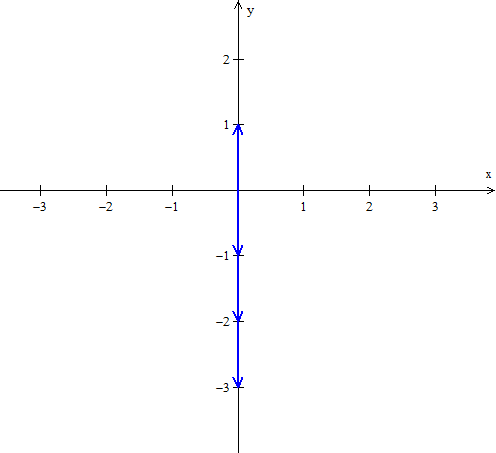Our graph represents the y - axis: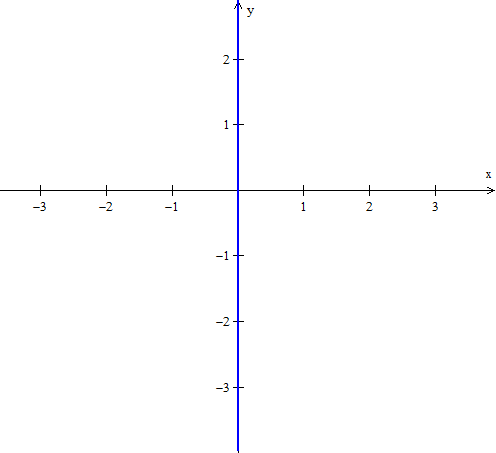Given g:R → R2 graph g(t) = (t,t3)
• Plotting a few values for t will give us an idea of the two dimensional graph.
• t = 0 yields g(0) = (0,03) = (0,0)
• t = 1 yields g(1) = (1,13) = (1,1)
• t = − 1 yields g( − 1) = ( − 1,( − 1)3) = ( − 1, − 1)
• t = 2 yields g(2) = (2,23) = (2,8)
• t = − 2 yields g( − 2) = ( − 2,( − 2)3) = ( − 2, − 8)
•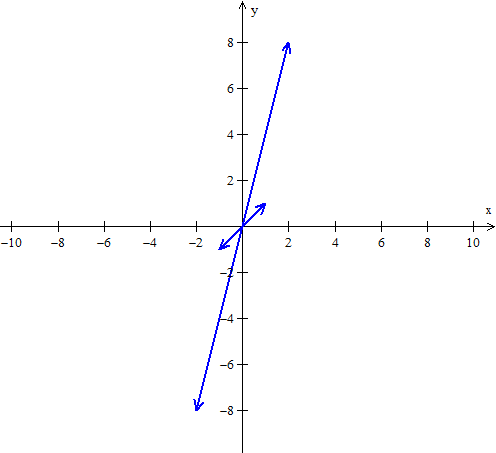Our graph represents the function y = x3: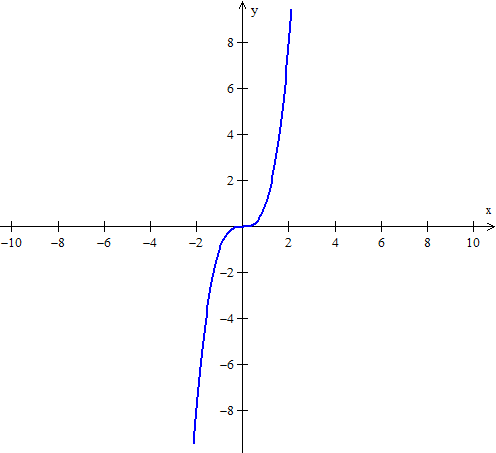Given h:R → R2 graph h(t) = (cos(t),sin(t))
• Plotting a few values for t will give us an idea of the two dimensional graph.
• t = 0 yields h(0) = (cos0,sin0) = (1,0)
• t = p yields h(p) = (cosp,sinp) = ( − 1,0)
• t = [p/2] yields h( [p/2] ) = (cos[p/2],sin[p/2]) = (0,1)
• t = [3p/2] yields h( [3p/2] ) = (cos[3p/2],sin[3p/2]) = (0, − 1)
•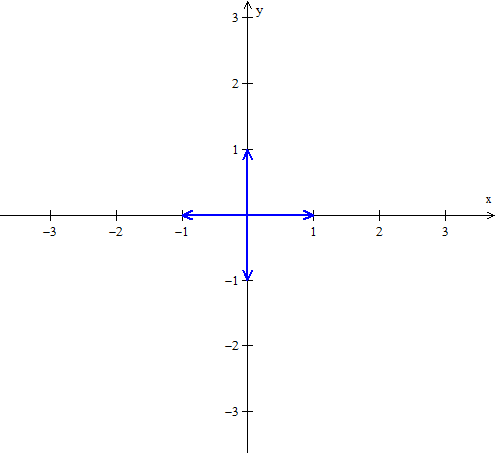Our graph represents the circle centered at the origin with radius 1: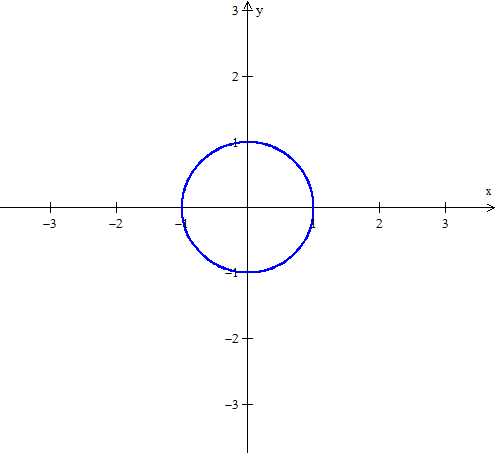Given f:R → R2 graph f(t) = (2,1)
Note that no matter what value of t we input, our point will always be (2,1), thus f maps the real line into a point in space.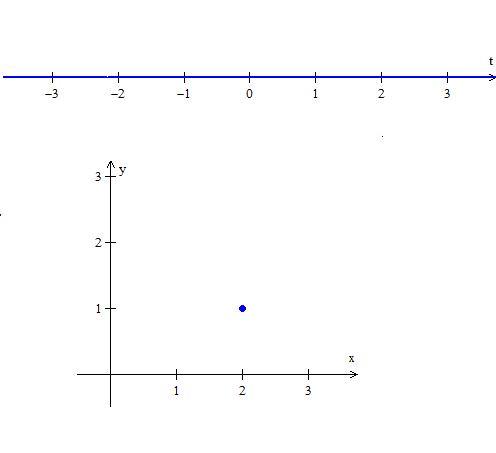Given f:R → R3 graph f(t) = (t,t + 1,t)
• Plotting a few values for t will give us an idea of the three dimensional graph.
• t = 0 yields f(0) = (0,0 + 1,0) = (0,1,0)
• t = 1 yields f(1) = (1,1 + 1,1) = (1,2,1)
• t = − 1 yields f( − 1) = ( − 1, − 1 + 1, − 1) = ( − 1,0, − 1)
• t = 2 yields f(2) = (2,2 + 1,2) = (2,3,2)
• t = − 2 yields f( − 2) = ( − 2, − 2 + 1, − 2) = ( − 2, − 1, − 2)
•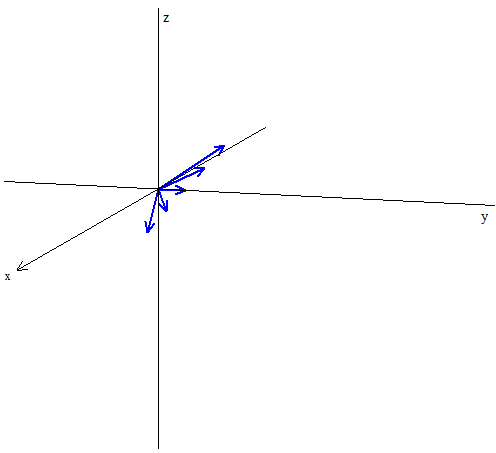Our graph represents a line: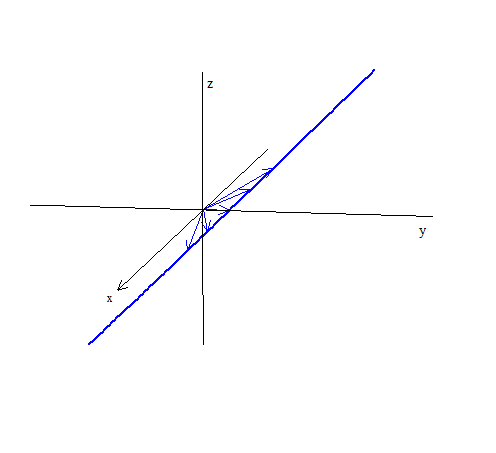Given f:R → R2, f(t) = (t2 + 1,t) find a function of x and y which represents the same graph.
• Let each component of the vector (t2 + 1,t) equate the x and y coordinates, that is x = t2 + 1 and y = t.
Substituting yields the rectangular expression x = y2 + 1.
Given f:R → R3, f(t) = (2t,t2 − 1,0) find a function of x, y and z which represents the same graph.
• Let each component of the vector (2t,t2 − 1,0) equate the x, y and z coordinates, that is x = 2t, y = t2 − 1 and z = 0.
Note that t = [x/2], so that substitution yields the rectangular expression y = ( [x/2] )2 − 1 = [(x2)/4] − 1.

*These practice questions are only helpful when you work on them offline on a piece of paper and then use the solution steps function to check your answer.

### Maps, Curves & Parameterizations

Lecture Slides are screen-captured images of important points in the lecture. Students can download and print out these lecture slide images to do practice problems as well as take notes while watching the lecture.

• Intro 0:00
• Maps, Curves and Parameterizations 1:10
• Recall
• Looking at y = x2 or f(x) = x2
• Departure Space & Arrival Space
• Looking at a 'Function' from ℝ to ℝ2
• Example 1
• Definition 1: Parameterized Curve
• Example 2
• Example 3

### Transcription: Maps, Curves & Parameterizations

Hello and welcome back to educator.com and welcome back to Multivariable Calculus.0000

We just finished our discussion of vectors and lines and planes and things like that.0005

Now we are going to actually start getting into the Multivariable Calculus.0009

Today's lesson, I am going to discuss a very important topic.0013

There are not going to be too many examples, but it is going to be mostly discussion.0015

It is going to be a global discussion about what we are going to be talking about when we talk about functions from one space to another.0020

So we are going to introduce this notion of a map.0029

Now you remember that we talked about parameterizing a line and the parameterization of a plane, now we are going to start parameterizing every single function that we deal with.0032

As it turns out, parameterization is a very powerful technique, much more powerful than the representations that you have been used to all of these years.0042

For example, if y = x2, y = x3, where you have the 1 independent variable and the dependent variable.0050

Now, we are going to take a little bit of a twist and have a different way of looking at that, a much broader, a much more general way of looking at that.0058

So, with that, let us go ahead and recall a few things and we will go ahead and jump right on in.0065

Let us recall a few things, in terms of notation.0072

What we are talking about is 1 space, basically just a real number line, that is what the R stands for.0077

This is 1 space.0087

Now it is my habit to actually notate R slightly differently.0090

I usually... in books you are going to see the real numbers with sort of these double lines, for the real numbers, the rational numbers, there is a specific notation, a letter representing these things.0096

So if I do it sometimes this way, or I do it sometimes that way, they mean the same thing.0108

It just means the real number line.0113

So we will do R2, that is 2 space.0115

In other words, what they are saying is you have 2 number lines.0120

R3, that is 3 space, you need 3 numbers to represent a point in 3 space, and of course RN that is the general.0125

That is just n space, so that is the most general dimensional space we can talk about.0137

Let us start by looking at the simple function y = x2, but let us look at it in a slightly different way.0144

Let us look at y = x2, or f(x) = x2, a slightly more general than this, but again this is how you are used to seeing it all of these years, this is how we have dealt with it.0150

We take a number, we do something with that number, and then we spit out a number that ends up being the f(x).0170

It ends up being the y value, and then we end up graphing this.0179

We are going to look at this in a slightly different way, I am going to draw two separate number lines.0181

I am going to draw one number line over here, then I am going to draw another number line over there.0187

Let me label this as 0, then we will do 1, then we will do 2 and we will do 3 and of course it goes on in both directions.0196

Over here let me go ahead and put 0, 1, 2, 3, 4, 5, 6, 7, 8, and 9.0201

Now, what f does, what this y = x2 does, we are going to take a value and some number, a real number, and we are going to do something to it.0215

We are going to square it and spit out another number.0226

Notice, we started in the real number line, the 1 space, and we have done something to it and then the number that we get back is still another number.0230

We end up back in the same space, or if you want to think of this as two separate spaces, it is probably the best way to do it.0240

In other words, this function takes a number... for example if I put 0 in there... it takes 0, it does something to it, and it ends up at 0 on another number line.0247

Consider this just another space but it happens to be the same space as the number from which you chose x.0260

If I take 1, and I put it in there, I square it and I end up getting, spitting out, 1.0265

If I take 2, I end up doing something to it, I spit out 4, and 3, I spit out 9, and so on in both directions negative and positive.0273

What we are doing is we are associating to each number in one space, in the real number line, we are associating another number in a copy of that space.0285

Again, the real number line. What we say is that f maps x to f(x).0298

In other words, we are mapping 0 to 0, 1 to 1, 2 to 4, 3 to 9, whatever the function happens to be.0307

This idea of a map, 2 separate spaces, in this case they happen to be the same, but they do not have to be.0314

That is what we are going to generalize in a minute, you can actually take a number in 1 space, do something to it, do a bunch of things to it, and actually end up in a completely different space all together.0321

You could end up in R2, R3, that is what we are going to do in a minute.0331

But we want to be able to see these functions that we have been dealing with in a different way.0336

We do not want to think of them as being y = x2, we want to think of it as taking a number x, doing something to it, and getting a number in a different space.0342

Again, in this particular example, the two spaces, the departure space, the arrival space, happen to be the same space.0352

Now, there is a notation for this.0362

This notation is actually going to be very important, it is going to be a notation we are going to be using often.0366

It is going to be ubiquitous throughout mathematics.0371

It is a very simple notation, but it is actually quite powerful when you speak generally about functions.0374

We say that f is a mapping from R to R.0382

Defined by x going to x2.0388

This is the general notion, what we are telling you is that f is a function that takes a number in the real number space, does something to it, and then spits out a number that also happens to be in the real number line.0394

A number in other words, not a vector. We actually give the definition of that, it means take x, and square it.0405

This is a pretty standard notation for a mapping, that is what it is.0411

You want to think of a function as a mapping, that is the most important thing.0417

Another way of looking at this, which I think is a little bit better, a little bit more pictorial way of looking at this is let us just say we have this space, and let us say we have this space.0422

They can be the same, they can not be the same.0433

We call the numbers that you choose, in other words, the independent variables, the x's that you choose randomly the departure space.0438

Once you do something to that particular number, whatever it is you are doing to it, however it is you are transforming it, we call it the arrival space.0446

So, this is... whoops, we are not going to have these random stray lines, we definitely do not want that especially when we are dealing with pictures here... let us close this off here... you know what, let me go ahead and do this on the next page, because I want you guys to see this really clearly.0457

It is the best way to consider this, so let me go ahead and erase that.0483

Let me move on to... there we go... so let me draw a little something like this, and a little something like this.0488

This is the departure space, it is the number that we choose.0498

This is the arrival space, so let us just pick a couple of random points.0504

So this is x1, x2, x3, so this is x1, x2, x3, and what we do, is when we take x1, do something to it, operate on the function, or use the function to operate on this... let us say this maps to here and this maps to here... we end up in certain places in the arrival space.0510

That place is in the arrival space, so this is a pictorial version of what it is that we are doing when we think of a map.0532

That is what is important, we are taking a number, we are doing something to it and we are ending up with another number, or vector, or could be anything.0537

It turns out in this particular case that the y = x2, this space happened to be the real number line, and this space also happened to be the real number line.0546

These are the functions we have been dealing with all of these years, ever since we were kids, and up through calculus.0556

But now, in multivariable Calculus, we want to become a little bit more sophisticated.0560

We want our spaces to become more sophisticated.0565

Now, what Descartes did was as far as what you know and what you have been dealing with as far as graphs are concerned, for example if you saw the y = x2 function, you are accustomed to seeing something like this.0569

What Descartes did was he took these two real number lines, this departure space and this arrival space and he set them perpendicular to each other, that is all he did.0584

So this is one space, and this is another space.0594

If you juxtapose them, because you can do that on a 2 dimensional sheet of paper, then if you map, you know... like... 1,1,2,4,3,9,4,16, the particular function, you end up getting this thing called a graph.0599

You get a curve in 2 space, that is what he did, that is where the notion of a graph came from.0612

What is really happening is you are mapping from one space to another space.0618

You are taking numbers from one space, doing something to them, and ending up in another space.0624

In this particular case, they both happen to be the same space, the real number line, R1.0628

Now, let us try looking at a function where now we are going to pick where our departure space is going to be the real number line.0636

Our arrival space is going to be 2-space, it is going to be 2-dimensional, let us see what that looks like.0644

Let us try looking at a function, and I will put function from R to R2, defined by the following: f(t) = t and t2.0655

Notice what I have done here.0686

This is vector notation, this is the x component, this is the y component.0690

If we are speaking in terms of x's and y's, so basically we have... we are taking a number, any number from the real number line and we are saying that what we are going to produce is a vector, a 2-vector, and the first component of that vector is just going to be the number t itself.0697

But, the second component is going to be t2.0716

This is a map, so I have taken a number, a real number from the number line and created a vector in R2.0720

Well a vector in R2 is a directed line segment, but it is also just a point, and the coordinates of that point are t and t2.0727

So what we are doing here is we are taking the real number line and we are taking numbers from the real number line and we are mapping them onto 2 space.0736

That is what we are doing.0750

So we might take the number 1, map it to 1 and 1, take the number 2, map it to 2 and 4, take the number 3 and map it to 3 and 9, and that is what we are going to do.0752

In fact let us do that for a couple of these so that we do a pictorial representation.0765

We are just taking numbers from the real number line, so here the departure space is the real numbers, and the arrival space is going to be R2.0773

We consider them 2 spaces, that is the idea.0782

So now, if I actually do this map, let me rewrite the map again, f(t) = t and t2.0787

Now let me go ahead and map a couple of these things.0800

Well if t =0, we will get 0,0.0802

If we do t=1, we will get 1 and 1.0808

If I map a 2, so it is going to be 2 and 4, so I will go to 2, go to 4.0813

If I take t=3, the point is going to be 3,9.0821

Notice what you get. You still end up with this parabola y = x2, but now it is not written as y = x2.0826

It is written as a map with a given parameter, that parameter t, you take that 1 parameter t and then the x... you actually map it to 2-space, well 2-space you need 2 numbers to represent a point, a vector in that space.0835

The first point is t, the second point is t2.0851

This is the power of parameterization, so this is a parameterization of the function y = x2, and this is a much more powerful way of looking at it.0856

What you have done here is this -- this is a vector, so (0,0) that is that vector, (1,1) that is that vector, (2,4) that is that vector, (3,9) that is that vector.0864

When you connect all of the endpoints of the vectors, you end up getting your curve in 2 space.0877

That is what we are doing.0885

Let us do an example and then we will give a formal definition of what we mean by a parameterized curve in n space.0890

So, example 1.0898

Let us say that f(t) = ... oh, let me use my notation so that you get used to seeing this... so f is a map from the real number line to 2-space.0903

Defined by f(t) = (cos(t),sin(t)), where t... and this particular case I am going to specify the interval t, I do not have to, I can just let it go, but what the heck, let us just go ahead and specify... so t goes from 0 all the way to 2pi.0920

If I take t = 0, and if I do... so that becomes, so t = 0, that is the point that I am pulling from the real number line, I am going to do something to it.0955

I am going to do this to it, and I am going to end up at (cos(0),sin(0)), which is (1,0).0968

So, I end up over here. That is my vector, that is my point in 2 space.0971

I am going to go through some other points.0976

Let us just take t = pi/4, a 45 degree angle, yeah pi/4.0979

Again, a radian measure is a real number, so pi/4, if I do (cos(t),sin(t)), I will get (1/sqrt(2), 1/sqrt(2)).0986

That will end up putting me over here.0999

If I take t = pi/2, now I am going to get (cos(pi/2),sin(pi/2)), that will put me at (0,1), so that will put me over there.1001

If I keep going like this, go all the way up to 2pi, these vectors that I get, that vector, that vector, that vector, that vector, that vector, if I connect all of the dots of these vectors, I am going to get the circle on the plane.1009

So this thing right here, this function is the parameterization of the unit circle in R2.1026

That is what we are doing. We are taking the parameter and deciding... we are creating curves in a space of a given dimension.1038

In this case, we ended up creating a curve in 2-space.1049

Now let us go ahead and give a formal definition of what it is we mean by a parameterized curve.1055

This one I am going to go ahead and move on to the next page.1059

It is going to be a slightly long definition, but hopefully we have notated it properly.1064

So, a parameterized curve... I am going to write it a little bit better here... a parameterized curve in RN, or n space, is a map... let us call it c... from R to RN, which to each point in R associates a vector, which is also a point, in RN.1070

When these vectors, or points, are connected, we get a curve in RN.1143

So as we saw in the previous example, we got a curve in 2-space.1167

So we are not thinking that it is a function anymore, the way we used to as far as y = x2.1173

You can do that, I mean you can certainly turn this parameterization into a y = x2, but now we want to sort of break away from that.1178

We want something a little more free, a little more general, a little more sophisticated, and you will see just how sophisticated in a bit when we start differentiating.1183

We want to think of it as, now let us say I do it in 3 space.1193

Let us say I take some value, some t, something from the real number line -- it is always going to be a number, the map is always going to be like this, the departure space for a curve, in n space, the departure space is always going to be the real number.1197

It is always going to be like this, for a curve in n space the departure space is always going to be the real number line.1212

The arrival space can be any dimensional space, R1, R2, R3.1218

In R3 you might have some random curve that goes all over the place. There is a way to parameterize that.1223

In other words there is some map, and of course for R2 and R3 we can visualize it, but again this is an algebraic definition.1229

Because it is an algebraic definition, it is very powerful.1236

We can think of a curve in 15 dimensional space, it is perfectly valid and we can work with it mathematically.1241

We may not be able to see it or visualize it, but it does exist, it is real. We can work with it.1247

Curve in RN and, I will say one more thing about that.1255

The coordinates of x -- of c, I am sorry -- the coordinates of c are functions of t.1261

You saw that already, so c(t) = some one function from... actually let me use f instead of x... It is going to be some function of t and then another function of t, and so on, depending on how many dimensions you are dealing with.1280

In the previous example, we had 2 functions of t, we had cos(t) and we had sin(t).1301

Again, a vector is made of components, those components are actual functions of t. This is the power of parameterization.1308

Let us look at another example here. Let us do a 3 space example.1318

So, example number 2. Let us let x(t) and we are going to be several different letters, x, y, sometimes c, occasionally I am going to be writing it with capital letters where I am not going to put the vector sign on top of that.1325

The notation is important, however the notation I am not going to... there are going to be different types of notation, but it will be consistent within the notation itself, in other words you will see the same arrangement, but we are just going to be using different things.1344

So, x(t) is a map from R to R3, so this time we are doing the curve in 3 space, defined by x(t) = (cos(t),sin(t),t).1358

Now, we have a curve in 3 space.1390

Let us see if we can find out what this curve looks like.1395

Let me go ahead and draw that, and that, and this.1398

This is the general representation of a 3 dimensional space, this is the x-axis, this is the y-axis, this is the z-axis.1403

We already did the cos(t),sin(t), we know that that is a circle, so in the x,y plane that is the circle.1412

In the x,y plane there is no z component, but now we have added a z-component.1420

For every value of t we actually not only move in a ... we do not just move in a circle... every time we go t, we actually go up a little bit, sort of like a ramp, a parking ramp... have you been to those parking ramps?1425

You are still moving in a circle as far as the x,y, coordinate is concerned, but every time you move, you are also going up.1441

What you will end up getting, the curve you are going to get, is a spiral.1447

That is exactly what it is, so this curve is going to end up being a spiral.1453

It still moves in a circle as far as the x,y plane is concerned, if you are looking down the z-axis it still looks like a circle, but now you have this third component t, the z-axis.1461

So, it is actually moving up as it turns. I hope that makes sense. It is going to look like this.1474

This t is changing, in other words t is also the z axis, there is a third component here.1480

This is a curve, so this is the parameterization of the unit spiral in R3.1485

That is the idea. If you are given a particular curve, you can parameterize it with some map that looks like this.1494

Then, when you have this map you can begin to play with it algebraically.1502

As you will see in the next lesson we will begin to start differentiating it and... well, yeah, other things too.1507

So, now, let us go one more up here, I will just do one more example.1515

Example number 3.1527

Let f be a map from R to R2, in other words a curve in 2-space, defined by f(t) = t and t2.1532

So this is the x-coordinate, right? This is the y-coordinate, it is a curve in the 2 space, this happens to be the parameterization of the parabola in the plane.1550

Now, let us go ahead and recover a function, let me see here, 1, 2... you know what, no, this is... well, that is okay we have already started it so let us go ahead and finish it.1560

So recover a function y in terms of x.1583

Now I have given you this parameterization, and we want to be able to recover some function the way we are used to seeing it, y as a function of x.1596

Dependent is something function of independent.1605

We are given the parameter t, the t is what is in common, so essentially all you have to do is eliminate the t from them and set y equal to some function of x.1608

So, x = t, and y = t2.1619

Well if x = t, let us go ahead and just put this x wherever we see a t.1624

So, = x2, there you go, that is it.1630

So you eliminate the t, the parameter, and you end up recovering some function of y = x2.1635

It becomes a lot more difficult... in fact I am not even sure, well... in 3 space and 4 space, but this is the power of parameterization.1641

We have been dealing with simple functions all these years simply because we are accustomed to dealing with 2 space and 3 space.1652

Our natural intuitive surroundings, you know, we have a sense of paper, we have a sense of flat paper.1656

We have a sense of 3-dimensional space, however things can obviously become a lot more complicated even when you are dealing in 3 space.1663

4-space, 5-space, 6-space, it becomes virtually impossible to deal with.1670

This is why we do parameterization, it is a much more sophisticated way of dealing with functions.1674

It also happens to work for lower dimensional cases, in other words R2.1680

Here is how you want to start thinking about functions.1687

Now, let us go ahead and recap here.1693

What we have done, as far as all of these years, what you have been working with are maps, or general functions, y = some function of x.1698

Those are maps from R to R, in other words, you take some value x, you do something to it, and you spit out another number. Numbers to numbers.1707

Today we have introduced the notion of curves, which are maps from the real number line, you take a number, and you create a vector to RN.1717

Well, we are also going to be going the other direction.1728

We are going to begin talking about functions in which you will take a vector, and we will do something to that vector and what we will spit out is a number.1731

To top it all off, we are eventually the most general is RN to RP, we are going to take a vector in n space, we are going to do something to it, and we are going to end up spitting out a vector in p space.1740

We are going to work from one certain dimensional space to another completely dimensional space.1758

This is the power of multivariable calculus, that you can actually do this, that you can actually do the calculus you know.1764

Everything that you learned in single variable calculus still applies and yet now it is going to become a little bit more sophisticated, more powerful.1770

It is really quite extraordinary.1779

So, next lesson we are going to start with the actual calculus in n space.1781

Until then, thank you for joining us at educator.com and take care, bye-bye.1785

OR

### Start Learning Now

Our free lessons will get you started (Adobe Flash® required).